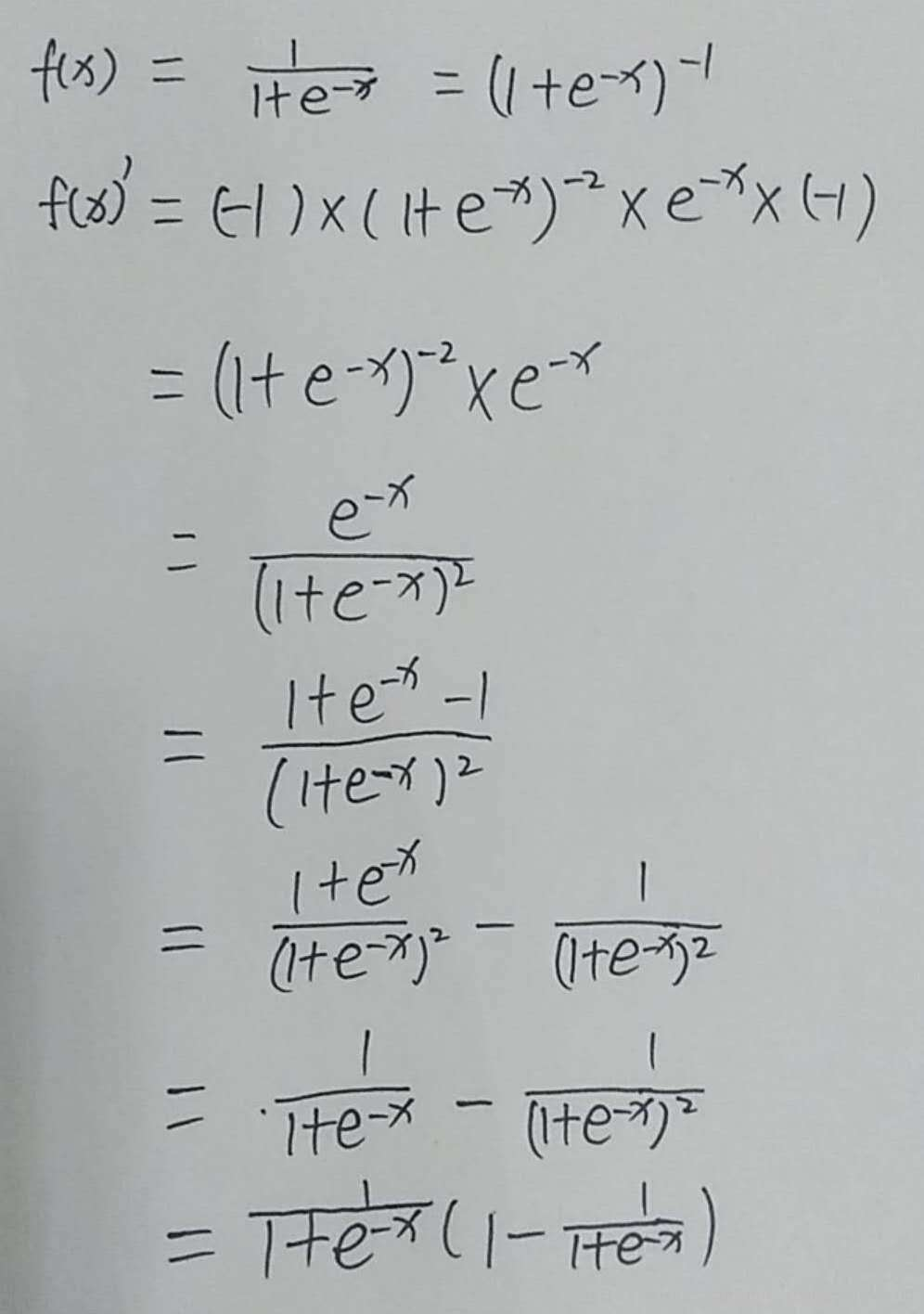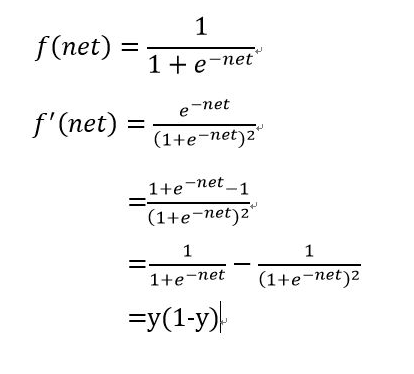• 回顾原由：在推荐系统中，排序阶段会用到逻辑回归，那自然要在温习下Sigmoid函数求导过程。Sigmoid函数：在对Sigmoid函数求导，还需回顾4个求导知识点(只有一点数学公式，不用紧张，你一定可以看懂)：  &...
回顾原由：在推荐系统中，排序阶段会用到逻辑回归，那自然要在温习下Sigmoid函数求导过程。Sigmoid函数：在对Sigmoid函数求导，还需回顾4个求导知识点(只有一点数学公式，不用紧张，你一定可以看懂)：    1、为什么要对Sigmoid函数求导？其实就是求极值。2、进行求导等于3、进行求导等于4、复合函数求导：f(g(x))' =，例如：，那么就是g(x)Sigmoid求导过程：’ =…………………………（1）两个负号抵消           =…………………………（2）加1和减1           =…………………………（3）           其中可以整体替换下为t(x)           = t(x)(1-t(x))                                       ……………………………（4）遇到一个小问题：的求导犯过错，也是复合函数求导，正确求导结果：*(-x)'=思考一个问题：为什么要对Sigmoid函数进行求导呢？在这里有什么用呢？
附上一个英文解释的很精细的求导过程：
https://towardsdatascience.com/derivative-of-the-sigmoid-function-536880cf918e


展开全文• sigmoid函数求导
sigmoid函数求导展开全文• sigmoid函数求导与自然指数
                在神经网络里经常使用sigmoid做激活函数，它的导数是怎么样求解呢？因为要使用它的导数来计算梯度下降。这个过程如下：1. sigmoid函数：f(z) = 1 / (1 + exp( − z))导数：f(z)' = f(z)(1 − f(z))求导过程如下：下文解释e的来由：https://betterexplained.com/articles/an-intuitive-guide-to-exponential-functions-e/1. TensorFlow API攻略http://edu.csdn.net/course/detail/44952. TensorFlow入门基本教程http://edu.csdn.net/course/detail/43693. C++标准模板库从入门到精通 http://edu.csdn.net/course/detail/33244.跟老菜鸟学C++http://edu.csdn.net/course/detail/29015. 跟老菜鸟学pythonhttp://edu.csdn.net/course/detail/25926. 在VC2015里学会使用tinyxml库http://edu.csdn.net/course/detail/25907. 在Windows下SVN的版本管理与实战  http://edu.csdn.net/course/detail/25798.Visual Studio 2015开发C++程序的基本使用 http://edu.csdn.net/course/detail/25709.在VC2015里使用protobuf协议http://edu.csdn.net/course/detail/258210.在VC2015里学会使用MySQL数据库http://edu.csdn.net/course/detail/2672            再分享一下我老师大神的人工智能教程吧。零基础！通俗易懂！风趣幽默！还带黄段子！希望你也加入到我们人工智能的队伍中来！https://blog.csdn.net/jiangjunshow


展开全文• 网上有不少关于sigmoid函数求导的文章（比如来自CSDN的博文sigmoid函数求导与自然指数），但是求导过程中对于怎么由(1/(1+exp(-x)))'一下推导至exp(-x)/(1+exp(-x))^2没有提及。虽然对于熟练函数求导的朋友们不难，...
• Sigmoid函数详细求导过程 Sigmoid函数求极值的两种方法 Sigmoid函数： 一，使用python包matplotlib绘制Sigmoid函数图形 1，python绘制Sigmoid图形代码如下： import numpy as np import matplotlib.pyplot as plt ...深度学习 keras nlp
• 持续汇总更新DNN层CNN层RNN层pooling层激活函数层：relu，sigmoid，常见的损失函数求导激活函数层神经网络中的激活函数有很多，比如sigmoid，relu等，对于激活层的反向传导算法，仅仅需要求出激活层的输出对输入的...softmax层
• 学习神经网络的反向传播过程中，涉及到对sigmoid函数进行求导、出来混总要还的。。丢掉的高数还是得捡起来，记录一下sigmoid函数的推导过程吧。 前置准备 求导的导数法则：若有g(x)=1f(x)g(x)=\frac{1}{f(x)}g(x)=f...神经网络 深度学习 机器学习
• 目录前言交叉熵损失函数交叉熵损失函数求导前言最近有遇到些同学找我讨论sigmoid训练多标签或者用在目标检测中的问题，我想写一些他们的东西，想到以前的博客里躺着这篇文章（2015年读研时机器学课的作业）感觉...
• 激活函数将非线性引入网络，因此激活函数自身也被称为非线性。神经网络是普适的函数逼近器，而深度神经网络基于反向传播训练，因此要求可微激活函数。反向传播在这一函数上应用梯度下降，以更新网络的权重。理解激活...
• sigmoid函数的导数是f′(x)=f(x)(1−f(x))​f ^ { \prime } ( x ) =f ( x ) ( 1 - f ( x ) ) ​f′(x)=f(x)(1−f(x))​ 推导过程如下： 1.先将f(x)稍微变形 f(x)=11+e−x=exex+1=1−(ex+1)−1​f ( x ) = \frac { 1 ...
• 损失函数用来评价模型的预测值和真实值不一样的程度，损失函数越好，通常模型的性能越好。不同的模型用的损失函数一般也不一样。损失函数分为经验风险损失函数和结构风险损失函数。经验风险损失函数指预测结果和实际...
• 作者 | Hugegene译者 | 刘畅责编 | Rachel出品 | AI科技大本营(id：rgznai100)【导语】本文对梯度函数和损失函数间的关系进行了介绍，并通过可视化方式进行了详细展示。另外，作者对三种常见的损失函数和两种常用的...
• 第 28节 - 逻辑回归之代价函数 (4)参考视频: 6 - 4 - Cost Function (11 min).mkv在这段视频中，我们要介绍如何拟合逻辑回归模型的参数θ。具体来说，我要定义用来拟合参数的优化目标或者叫代价函数，这便是监督学习...
• 2sigmoid函数求导3神经网络损失函数求导 1、sigmoid函数 ​ sigmoid函数，也就是s型曲线函数，如下：  函数：f(z)=11+e−z 导数：f′(z)=f(z)(1−f(z)) ​ 上面是我们常见的...
• 2sigmoid函数求导 3神经网络损失函数求导 1、sigmoid函数​ sigmoid函数，也就是s型曲线函数，如下： 函数：f(z)=11+e−z 函数：f(z)=\frac{1}{1+e^{-z}} 导数：f′(z)=f(z)(1−f(z)) 导数：f'(z)=f(z)(1-f(z)) ​ ...深度学习...